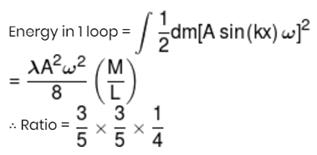Courses

# JEE Main Physics Mock - 5

## 25 Questions MCQ Test Mock Test Series for JEE Main & Advanced 2021 | JEE Main Physics Mock - 5

Description
This mock test of JEE Main Physics Mock - 5 for JEE helps you for every JEE entrance exam. This contains 25 Multiple Choice Questions for JEE JEE Main Physics Mock - 5 (mcq) to study with solutions a complete question bank. The solved questions answers in this JEE Main Physics Mock - 5 quiz give you a good mix of easy questions and tough questions. JEE students definitely take this JEE Main Physics Mock - 5 exercise for a better result in the exam. You can find other JEE Main Physics Mock - 5 extra questions, long questions & short questions for JEE on EduRev as well by searching above.
QUESTION: 1

### Emission of electrons in the photoelectric effect is possible, if

Solution: The wavelength should be low because lower the wavelength higher the frequency and for photo electric effect to occur we need a threshold frequency.
QUESTION: 2

Solution:
QUESTION: 3

### The acceleration due to gravity at a height 1/10th of the radius of the earth above the earth's surface is 8.2 m-s⁻1 Its value at a point at the same distance below the surface of the earth is

Solution:
QUESTION: 4
According to Hooke's law of elasticity, if stress is increased, the ratio of stress to strain
Solution:
QUESTION: 5
A 5 $\mu$F capacitor is fully charged across a 12V battery. It is then disconnected from the battery and connected to an uncharged capacitor. If the voltage across the capacitor becomes 3V, the capacitance of the uncharged capacitor is
Solution:
C1 * V1 = Q1. [Eq. 1]
Where, C1 = 5uF, V1= 12V.
This means capacitor 1 is able to gather up to 60uC of charge; before the circuit reaches voltage equilibrium and no more current flow is possible.
Now we transfer this bank of charge to another circuit with an uncharged capacitor, C2, which absorbs enough charge and leaves the circuit in equilibrium at 3V.
i.e. V2 = 3V
At 3V C1 can retain a charge of 15uC [using Eq. 1]. This means C2 most possess the remaining 45uC[Q2] of charge; at 3V[V2].
C2 = Q2/V2
C2= 45uC/3V
C2= 15uF
QUESTION: 6
If the error in the measurment of radius of a sphere is 2% , then the error in the determination of volume of the sphere will be
Solution:
QUESTION: 7

The volume of a gas will be double of what it is at 0oC (pressure remaining constant) at

Solution:
QUESTION: 8

The electric potential at any point (x,y,z) in a space is given by: V=4x2 volt. The electric field at (1,0,2) m in space is

Solution: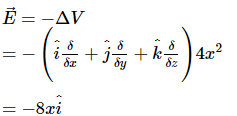Magnitude of electric field at the point (1m, 0, 2m) = − 8 V ∕ m

QUESTION: 9

An L.C. circuit contains 10 mH inductor and a 25 μ F capacitor. The resistance of the circuit is negligible. The energy stored in the circuit is completely magnetic at time (in milli seconds) being measured from the instant when the circuit is closed

Solution:

When R is negigible,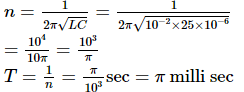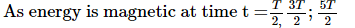Therefore, t = 1.57, 4.71, 7.85 ... milli sec

QUESTION: 10

The horizontal range of a projectile is R, when the angle of projection is 30o. The value of another angle of projection, for the same range, is

Solution:
QUESTION: 11
The first atomic reactor was constructed by
Solution:
QUESTION: 12
In the following question, a Statement of Assertion (A) is given followed by a corresponding Reason (R) just below it. Read the Statements carefully and mark the correct answer-
Assertion(A): A changing magnetic field acts as a source of electric field known as induced electric field.
Reason(R): Induced electric field can be produced by any static charge distribution.
Solution: A charged particle moving without acceleration produces an electric well as a magnetic field. It produces an electric field because it's a charge particle. But when it is at rest, it doesn't produce a magnetic field.So, when there will be no any magnetic field then not produce induced E.F.
QUESTION: 13
In the following question, a Statement of Assertion (A) is given followed by a corresponding Reason (R) just below it. Read the Statements carefully and mark the correct answer-
Assertion(A): Torque is an axial vector and directed along the axis of rotation.
Reason(R): Torque is equal to vector product of force to the position vector.
Solution:
QUESTION: 14
The astronomical telescope consists of objective and eye-piece. The focal length of the objective is
Solution:
QUESTION: 15
Frequency range of audible sound is
Solution:
QUESTION: 16
A stretched sonometer wire is in unison with a tuning fork of frequency f'. When the length of wire is increased by 2%, the number of beats produced per second is 5. The frequency of fork is
Solution:
QUESTION: 17
Bright colours exihibited by spider's web exposed to sun light are due to
Solution:
QUESTION: 18

If a ball of 80 kg mass hits an ice cube and temperature of ball is 100oC, how much ice becomes water? Specific heat of ball is 0.2 cal/gram.

Solution:
QUESTION: 19
A bullet of mass 50 gram is fired from a 5 kg gun with a velocity of 1 km/sec. The speed of recoil of the gun is
Solution:
QUESTION: 20

Two uncharged identical capacitors A and B, each of capacitance C, and an inductor of inductance L are arranged as shown in the adjacent figure. At t = 0, the switch S1 is closed while switch S2 remains open. At time t = t0=√LCπ/2, switch S2 is closed while switch S1 is opened.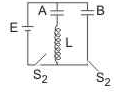Q. The charge on capacitor A after time t0 is

Solution:
*Answer can only contain numeric values
QUESTION: 21

A massless metal plate is placed on a horizontal tabletop lubricated with oil. The sheet is a square of side length ℓ = 1.0m and the oil layer has thickness h = 1.0 mm. Initially one edge of the sheet coincides with one edge of the table. The sheet is pulled outwards without rotation with a constant force F = 15 N. If coefficient of viscosity of the oil is η = 0.2 N-s/m2, how long (in second) will it take to pull half of the sheet out of the table?

Solution: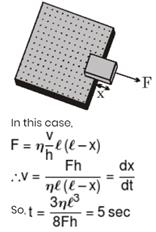*Answer can only contain numeric values
QUESTION: 22

Write the value of current i3 (in mA) in the circuit shown in the figure.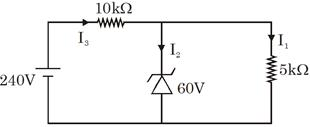Solution:

5 × 103 I1 = 60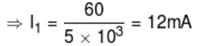Potential difference across
10 kΩ = 240 – 60 = 180 V
So, I3 × 10 × 103 = 180
⇒ I3 = 18 mA
I2 = I3 – I1 = 6 mA

*Answer can only contain numeric values
QUESTION: 23

A monochromatic light of wavelength 6500Å is used in YDSE. Now both slits are covered by two thin slab of refractive index 3.2 and 2.7 respectively. By doing so central bright fringe shifts to original 7th bright fringe. If both slabs have same thickness then thickness of slab is (in µm).

Solution: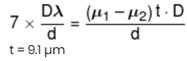*Answer can only contain numeric values
QUESTION: 24

A hollow sphere (mass m, radius R) is put inside a hollow cone (mass m, radius 3R and semi-angle 30°) as shown in the figure. The whole arrangement is rigidly fixed to the ground at the apex of the cone. Both the sphere and the cone are made of the same material (α = 10–4 /°C). Considering that major changes in dimensions could happen only due to heating effects, find the increase in potential energy (in Joule) of the system if it's temperature is increased by 100°C.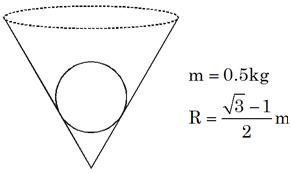Solution: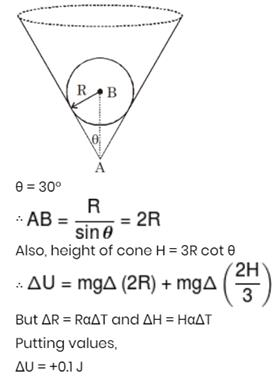*Answer can only contain numeric values
QUESTION: 25

A rod is clamped at both of its ends and stationary longitudinal waves are produced in it. In first experiment, the rod has a total of 4 nodes with amplitude of each anti-node to be 1 mm. In other experiment, the rod has a total of 6 nodes with amplitude of each anti node to be 2mm. The energy of vibrations in the rod in two cases is E1 and E2 respectively. Write value of E1/E2.

Solution: